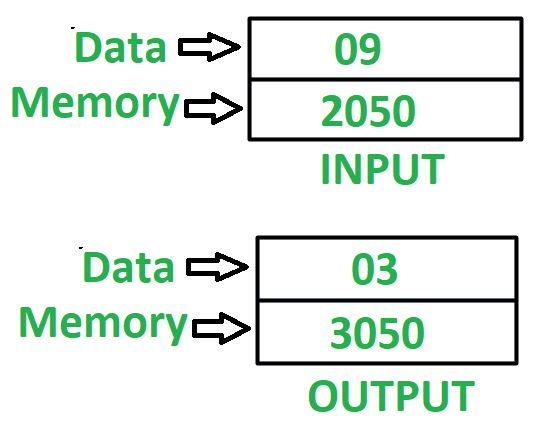Open in App
Not now

# 8085 program to find square root of a number

• Difficulty Level : Basic
• Last Updated : 28 Jun, 2020

Problem – Write an assembly language program in 8085 microprocessor to find square root of a number.

Example –Assumptions –
Number, whose square root we need to find is stored at memory location 2050 and store the final result in memory location 3050.

Algorithm –

1. Assign 01 to register D and E
2. Load the value, stored at memory location 2050 in accumulator A
3. Subtract value stored at accumulator A from register D
4. Check if accumulator holds 0, if true then jump to step 8
5. Increment value of register D by 2
6. Increment value of register E by 1
8. Move value stored at register E in A
9. Store the value of A in memory location 3050

Program –

2000 MVI D, 01 D <- 01
2002 MVI E, 01 E <- 01
2004 LDA 2050 A <- M
2007 SUB D A <- A – D
2008 JZ 2011 Jump if ZF = 0 to memory location 2011
200B INR D D <- D + 1
200C INR D D <- D + 1
200D INR E E <- E + 1
2011 MOV A, E A <- E
2012 STA 3050 A -> M
2015 HLT END

Explanation – Registers used A, D, E:

1. MVI D, 01 – initialize register D with 01
2. MVI E, 01 – initialize register E with 01
3. LDA 2050 – loads the content of memory location 2050 in accumulator A
4. SUB D – subtract value of D from A
5. JZ 2011 – make jump to memory location 2011 if zero flag is set
6. INR D – increments value of register D by 1. Since it is used two times, therefore value of D is incremented by 2
7. INR E – increments value of register E by 1